# IIC or NOT IIC? That is the Question!

By Sara Vance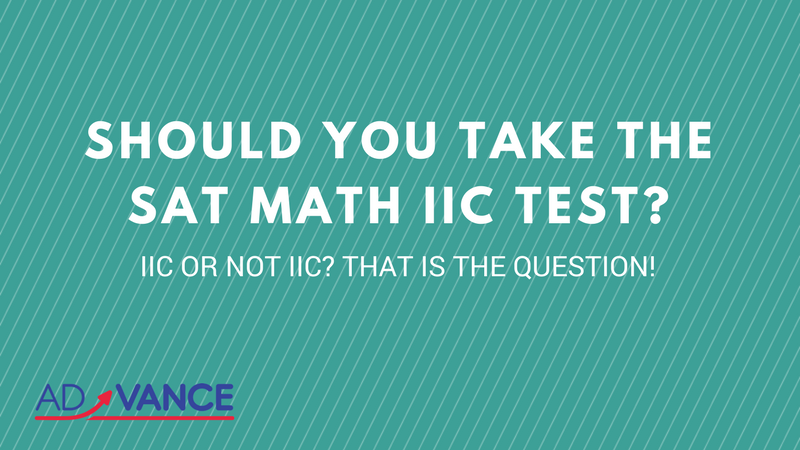While many students are very hesitant to take the Math IIC subject test because they fear it is too difficult, the reality is that students should find the MATH IIC no more intimidating than either the SAT I Math or the ACT.  Why you ask?

First, the Math IIC is a much more straightforward test: the questions are more direct and formula-based than those on the SAT I. They do not require you to do as much analytical thinking in order to get the answer.

If you know the formula being tested or the necessary math procedure, more often than not you can get the right answer. In addition, there are many questions which are simply calculator-driven.

Secondly, unlike the math sections of the SAT I and the ACT, the Math IIC docks you for guessing. Therefore, your score is determined by how aggressively or conservatively you take the test, in addition to the number of correct responses, thereby giving you TWO ways to raise your score!

If you have a general idea of the number of questions you should be leaving blank, that understanding can result in score increases of as much as 50-60 points.

Thirdly, the student is not expected to have learned every concept that the Math IIC tests. Many high schools do not teach everything that is on the test, such as vectors, parametrics, and polar coordinates, all of which are easy to learn.

Therefore, the student that takes the time to learn these concepts will have an advantage over those students who go into this test assuming that they have been taught everything that is being tested.  Such is not true of the SAT I math and the ACT math test: for the most part, these tests cover the basic concepts taught in high school math courses.

Finally, it is important that students understand that a subject test score is not the equivalent of a math or verbal SAT I score. The percentiles are what are important in evaluating your performance.

For example, scoring an 800 on the SAT I Math puts you in the 98-99+ percentile, but scoring an 800 on the Math IIC puts you in the 91st percentile. Similarly, a 750 Math IIC is in the 79th percentile, while a 750 Math SAT I is in the 92-95th percentile.

However, because the scope of concepts tested on the Math IIC is much narrower than that of a U.S. History or World History subject test, it is easier to prep for the Math IIC, so you might be able to score higher on it than you would on some of the other subject tests.

So what does it take for you to do well on the Math IIC test? Know the Math IIC Formulas and Procedures!

# WANT TO SCORE 700 OR HIGHER?

## KNOW THE SAT MATH 2C FORMULAS & PROCEDURES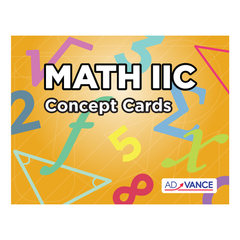The easiest way
to know all of the Math IIC formulas and procedures is by mastering the AdVANCE Math IIC flashcards in combination with . The flashcards have helped hundreds of students dramatically raise their scores!

# THE SAT MATH IIC FORMULAS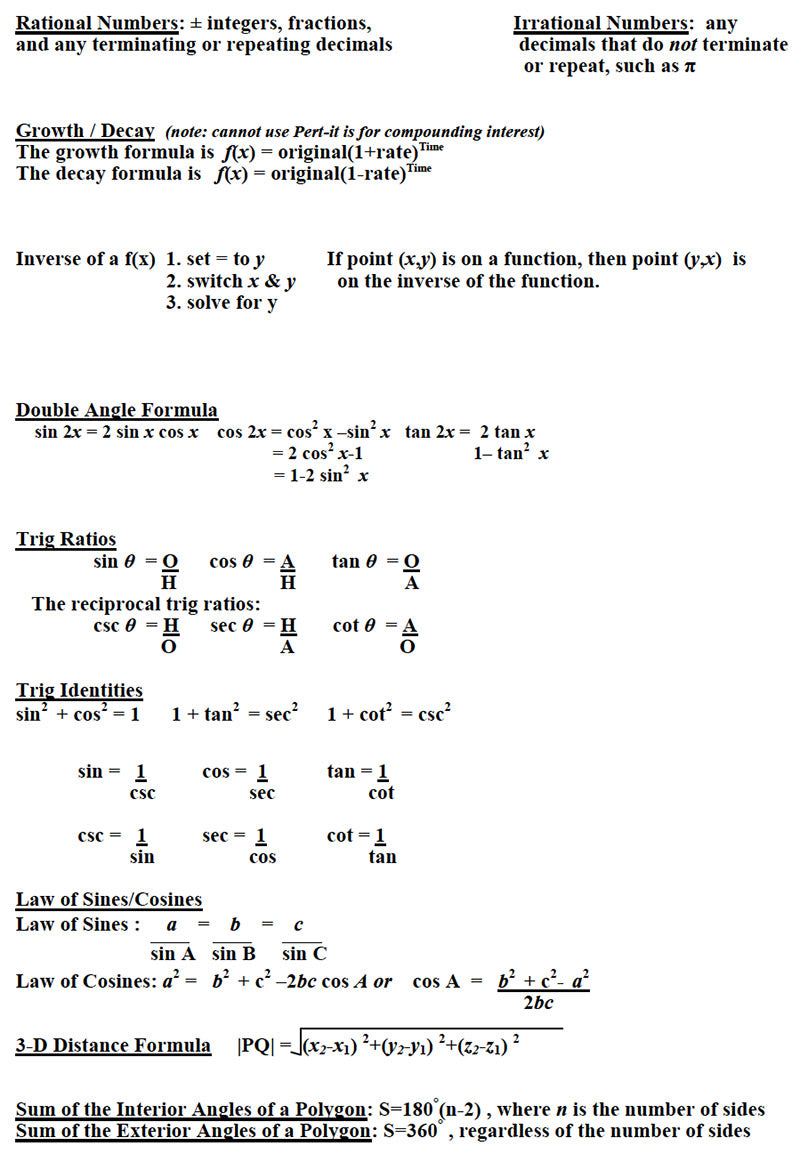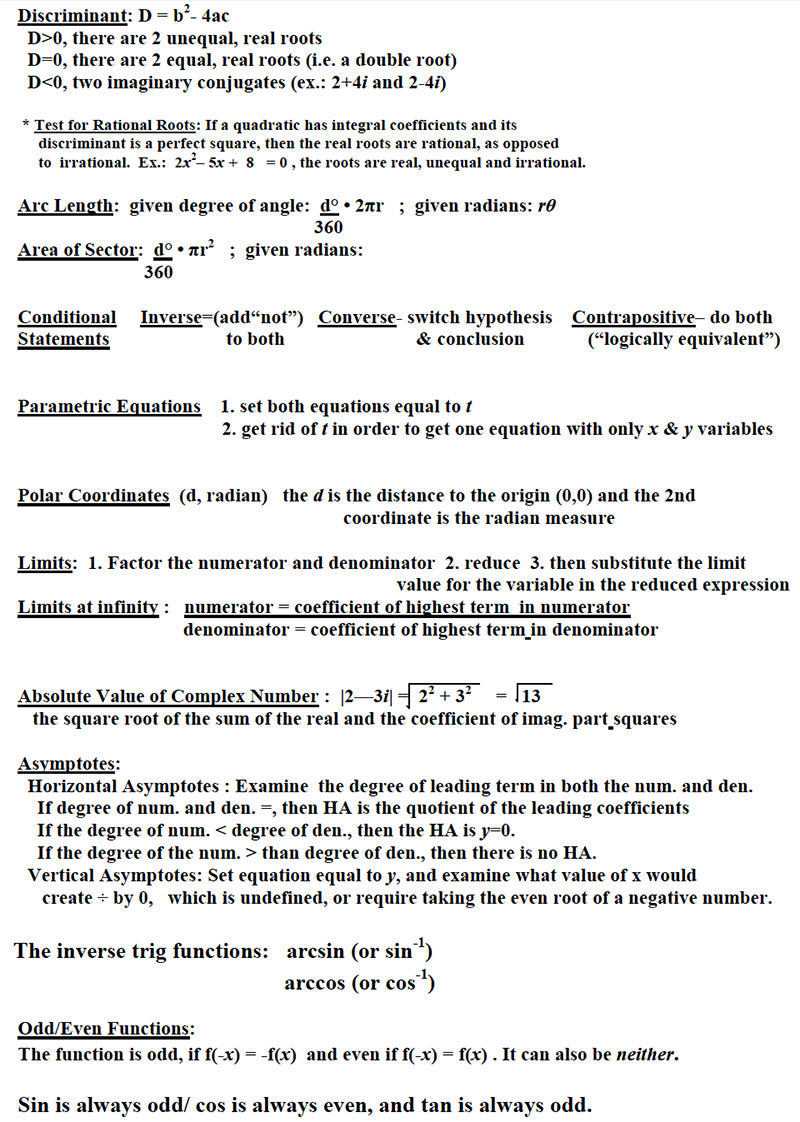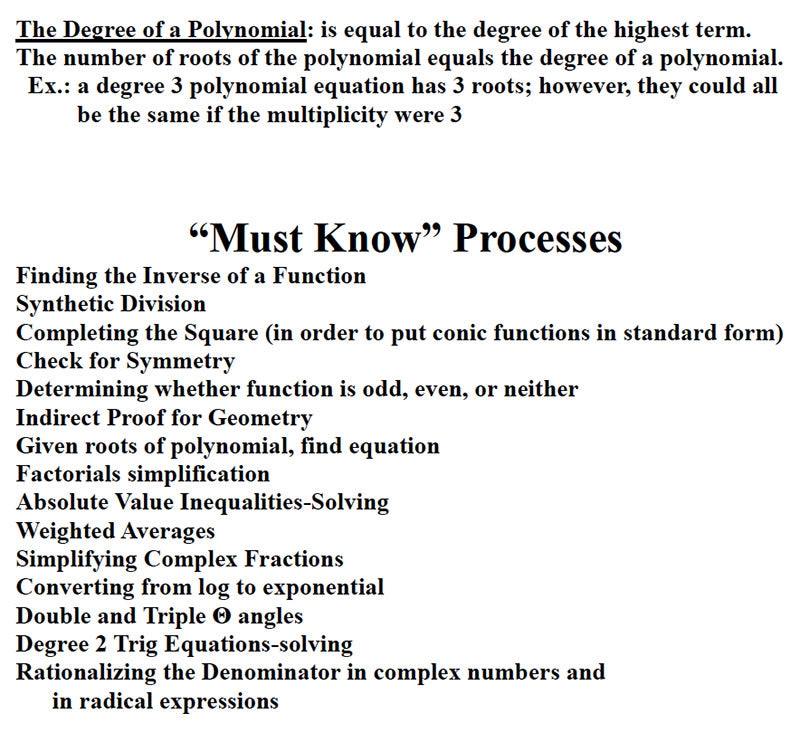Ok, now the rest is up to you. GOOD LUCK! If we can answer any questions, feel free to contact us.

# WANT TO SCORE 700 OR HIGHER?

## KNOW THE SAT MATH 2C FORMULAS & PROCEDURESThe easiest way
to know all of the Math IIC formulas and procedures is by mastering the AdVANCE Math IIC flashcards in combination with . The flashcards have helped hundreds of students dramatically raise their scores!